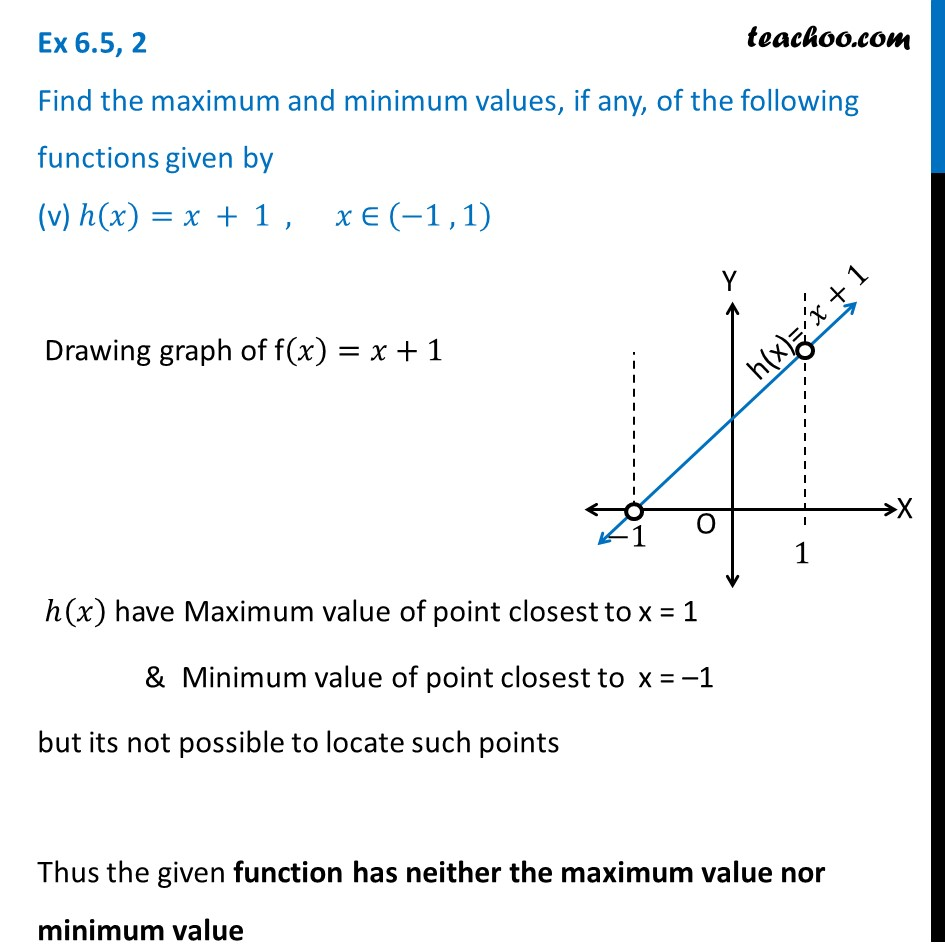1. Chapter 6 Class 12 Application of Derivatives (Term 1)
2. Serial order wise
3. Ex 6.5

Transcript

Ex 6.5, 2 Find the maximum and minimum values, if any, of the following functions given by (v) ℎ(𝑥)=𝑥 + 1 , 𝑥 ∈ (−1 , 1) Drawing graph of f(𝑥)=𝑥+1 ℎ(𝑥) have Maximum value of point closest to x = 1 & Minimum value of point closest to x = –1 but its not possible to locate such points Thus the given function has neither the maximum value nor minimum value